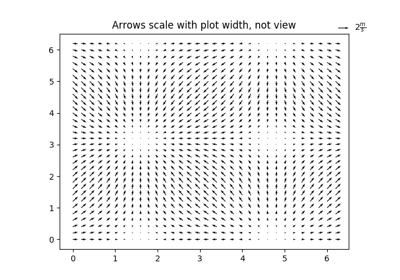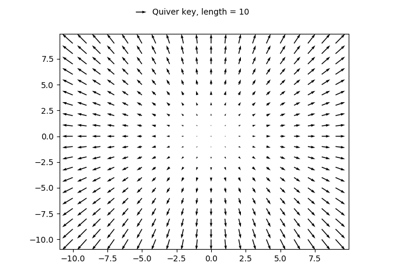# matplotlib.axes.Axes.quiverkey¶

Axes.quiverkey(Q, X, Y, U, label, **kw)[source]

Add a key to a quiver plot.

Call signature:

quiverkey(Q, X, Y, U, label, **kw)


Arguments:

Q:
The Quiver instance returned by a call to quiver.
X, Y:
The location of the key; additional explanation follows.
U:
The length of the key
label:
A string with the length and units of the key

Keyword arguments:

angle = 0
The angle of the key arrow. Measured in degrees anti-clockwise from the x-axis.
coordinates = [ 'axes' | 'figure' | 'data' | 'inches' ]
Coordinate system and units for X, Y: 'axes' and 'figure' are normalized coordinate systems with 0,0 in the lower left and 1,1 in the upper right; 'data' are the axes data coordinates (used for the locations of the vectors in the quiver plot itself); 'inches' is position in the figure in inches, with 0,0 at the lower left corner.
color:
overrides face and edge colors from Q.
labelpos = [ 'N' | 'S' | 'E' | 'W' ]
Position the label above, below, to the right, to the left of the arrow, respectively.
labelsep:
Distance in inches between the arrow and the label. Default is 0.1
labelcolor:
defaults to default Text color.
fontproperties:
A dictionary with keyword arguments accepted by the FontProperties initializer: family, style, variant, size, weight

Any additional keyword arguments are used to override vector properties taken from Q.

The positioning of the key depends on X, Y, coordinates, and labelpos. If labelpos is 'N' or 'S', X, Y give the position of the middle of the key arrow. If labelpos is 'E', X, Y positions the head, and if labelpos is 'W', X, Y positions the tail; in either of these two cases, X, Y is somewhere in the middle of the arrow+label key object.

## Examples using matplotlib.axes.Axes.quiverkey¶Demonstration of advanced quiver and quiverkey functionsQuiver Simple Demo